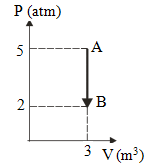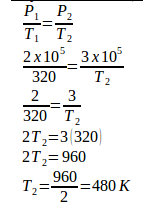# Isochoric thermodynamics processes – problems and solutions

1. PV diagram below shows an ideal gas undergoes an isochoric process. Calculate the work is done by the gas in the process AB.Solution :

Process AB is an isochoric process (constant volume). The volume is constant so that no work is done by the gas.

.[irp]

2. Three moles of monoatomic gas at 47oC and at pressure 2 x 105 Pa, undergoes isochoric process so that pressure increases 3 x 105 Pa. The change in internal energy of the gas is… Universal gas constant (R) = 8.315 J/mol.K

Known :

Initial temperature (T1) = 47oC + 273 = 320 K

Initial pressure (P1) = 2 x 105 Pa

Final pressure (P2) = 3 x 105 Pa

Universal gas constant (R) = 8.315 J/mol.K

Number of moles (n) = 3

Wanted: The change in internal energy of the gas.

Solution :

In the isochoric process, the volume is kept constant so that no work is done by the gas (W = 0).

ΔU = Q-W

ΔU = Q-0

ΔU = Q

ΔU = internal energy, Q = heat

Internal energy of gas :

ΔU = 3/2 n R ΔT = 3/2 n R (T2 – T1)The change in internal energy of gas :

ΔU = 3/2 n R (T2 – T1) = 3/2 (3)(8.315)(480-320)

ΔU = 3/2 (24.945)(160) = 3/2 (3991.2)

ΔU = 5986.8 Joule

[irp]

3. 0.2 moles of monatomic gases at 27oC are in a closed container. The heat is added to the gas so that temperature of gas becomes 400 K isUniversal gas constant (R) = 8.315 J/mol.K

Known :

Number of moles (n) = 0.2 mol

Initial temperature (T1) = 27oC + 273 = 300 K

Final temperature (T2) = 400 K

Universal constant gas (R) = 8.315 J/mol.K

Wanted : Heat is added (Q)

Solution :

In isochoric process, volume is kept constant so that no work is done by the gas (W = 0).

The first law of thermodynamics :

ΔU = Q-W

ΔU = Q-0

ΔU = Q

ΔU = internal energy, Q = heat

The internal energy of gas :

ΔU = 3/2 n R ΔT = 3/2 n R (T2 – T1)

ΔU = 3/2 (0.2)(8.315)(400-300)

ΔU = 3/2 (0.2)(8.315)(100)

ΔU = 249.45 Joule